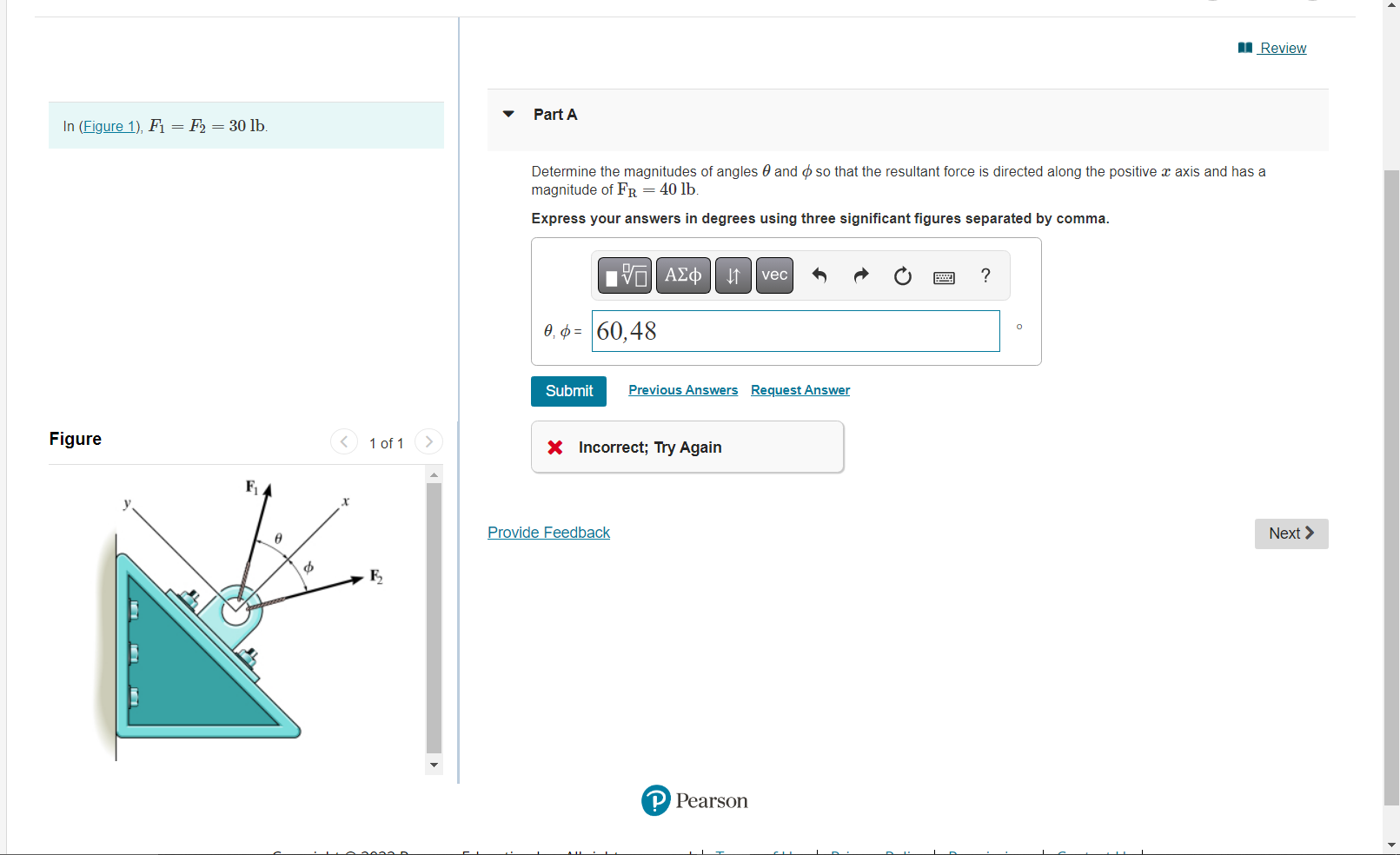# Question Solved1 Answer In (Figure 1), F₁ = F₂ = 30 lb. Figure F₁ $1 of 1 Ę₂ Review Part A Determine the magnitudes of angles and so that the resultant force is directed along the positive x axis and has a magnitude of FR = 40 lb. Express your answers in degrees using three significant figures separated by comma. 17| ΑΣΦ ↓↑ vec ? 0,= 60,48 Submit Previous Answers Request Answer X In (Figure 1), F₁ = F₂ = 30 lb. Figure F₁$ 1 of 1 Ę₂ Review Part A Determine the magnitudes of angles and so that the resultant force is directed along the positive x axis and has a magnitude of FR = 40 lb. Express your answers in degrees using three significant figures separated by comma. 17| ΑΣΦ ↓↑ vec ? 0,= 60,48 Submit Previous Answers Request Answer X Incorrect; Try Again Next > Provide Feedback P PearsonV8QGFB The Asker · Civil EngineeringTranscribed Image Text: In (Figure 1), F₁ = F₂ = 30 lb. Figure F₁ $1 of 1 Ę₂ Review Part A Determine the magnitudes of angles and so that the resultant force is directed along the positive x axis and has a magnitude of FR = 40 lb. Express your answers in degrees using three significant figures separated by comma. 17| ΑΣΦ ↓↑ vec ? 0,= 60,48 Submit Previous Answers Request Answer X Incorrect; Try Again Next > Provide Feedback P Pearson More Transcribed Image Text: In (Figure 1), F₁ = F₂ = 30 lb. Figure F₁$ 1 of 1 Ę₂ Review Part A Determine the magnitudes of angles and so that the resultant force is directed along the positive x axis and has a magnitude of FR = 40 lb. Express your answers in degrees using three significant figures separated by comma. 17| ΑΣΦ ↓↑ vec ? 0,= 60,48 Submit Previous Answers Request Answer X Incorrect; Try Again Next > Provide Feedback P Pearson